JEE  >  VITEEE Maths Test - 5

# VITEEE Maths Test - 5

Test Description

## 40 Questions MCQ Test VITEEE: Subject Wise and Full Length MOCK Tests | VITEEE Maths Test - 5

VITEEE Maths Test - 5 for JEE 2023 is part of VITEEE: Subject Wise and Full Length MOCK Tests preparation. The VITEEE Maths Test - 5 questions and answers have been prepared according to the JEE exam syllabus.The VITEEE Maths Test - 5 MCQs are made for JEE 2023 Exam. Find important definitions, questions, notes, meanings, examples, exercises, MCQs and online tests for VITEEE Maths Test - 5 below.
Solutions of VITEEE Maths Test - 5 questions in English are available as part of our VITEEE: Subject Wise and Full Length MOCK Tests for JEE & VITEEE Maths Test - 5 solutions in Hindi for VITEEE: Subject Wise and Full Length MOCK Tests course. Download more important topics, notes, lectures and mock test series for JEE Exam by signing up for free. Attempt VITEEE Maths Test - 5 | 40 questions in 50 minutes | Mock test for JEE preparation | Free important questions MCQ to study VITEEE: Subject Wise and Full Length MOCK Tests for JEE Exam | Download free PDF with solutions
 1 Crore+ students have signed up on EduRev. Have you?
VITEEE Maths Test - 5 - Question 1

### the area of the ellipse x2/a2 + y2/b2 = 1 is

VITEEE Maths Test - 5 - Question 2

### The equation of the tangent to the curve (1 + x2)y = 2 - x where it crosses x-axis is

VITEEE Maths Test - 5 - Question 3

### Find the value of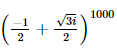VITEEE Maths Test - 5 - Question 4
If z₁,z₂ and z₃ are complex number such that
|z₁|=|z₂|=|z₃|=|1/z₁ + 1/z₂ + 1/z₃|=1,then |z₁ + z₂ + z₃ | is
VITEEE Maths Test - 5 - Question 5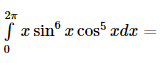VITEEE Maths Test - 5 - Question 6

If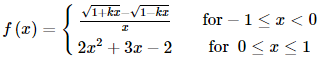is continuous at x = 0, then k =

VITEEE Maths Test - 5 - Question 7

The value of determinant ∆ of 3rd order is 9 then the value of ∆′2 where ∆′ is a determinant formed by cofactors of the element of ∆ is

VITEEE Maths Test - 5 - Question 8

The real part of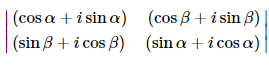is

VITEEE Maths Test - 5 - Question 9

Let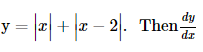at x = 2

is

VITEEE Maths Test - 5 - Question 10

The order of the differential equation x2+y2+2gx+2fy+c=0, is

VITEEE Maths Test - 5 - Question 11

The solution of the differential equation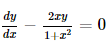is

Detailed Solution for VITEEE Maths Test - 5 - Question 11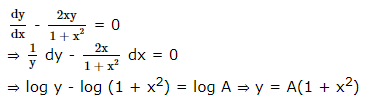VITEEE Maths Test - 5 - Question 12

Integrating factor of differential equation cos x dy/dx+y sin x = 1 is

VITEEE Maths Test - 5 - Question 13

The solution of the differential equation x2 dy/dx-xy=1+cos y/x is

VITEEE Maths Test - 5 - Question 14

If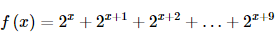, then find f′ 2

VITEEE Maths Test - 5 - Question 15

Set A has 3 elements and set B has 4 elements. The number of injections that can be defined from A into B is

VITEEE Maths Test - 5 - Question 16

If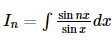where n > 1 , then In − In−2

VITEEE Maths Test - 5 - Question 17

∫[(x+sinx)/(1+cosx)] dx =

VITEEE Maths Test - 5 - Question 18

If cos-1(x/a)+cos-1(y/b)=α, then (x2/a2)-(2xy/ab)cosα+(y2/b2)=

VITEEE Maths Test - 5 - Question 19

Obtain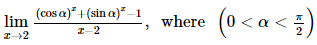VITEEE Maths Test - 5 - Question 20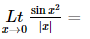VITEEE Maths Test - 5 - Question 21

The statement "If x is divisible by 8, then it is divisible by 6" is false if x equals

Detailed Solution for VITEEE Maths Test - 5 - Question 21

A sentence in "If....then..." form is called an implication.
The only time when an implication is false is when the "If" part of the sentence is true and the "then" part of the sentence is false.
The number 32 makes the first part of the statement true and the second part of the statement false.

VITEEE Maths Test - 5 - Question 22

If the value of a determinants is 11, then the square of the determinant formed by its cofactor will be

VITEEE Maths Test - 5 - Question 23

If A is an 3x4 matrix and B is a matrix such that A'B and BA' both are defined, then size of B is

VITEEE Maths Test - 5 - Question 24

In a 12 storey building 3 persons enter a lift cabin. It is known that they will leave the lift at different storeys. In how many ways can they do so if the lift does not stop at the second storey.

Detailed Solution for VITEEE Maths Test - 5 - Question 24

There are 10 storeys for three persons for leaving the lift
(these are other than second storey and one at which they enter the lift).
So required number is 10 P 3 = 720

VITEEE Maths Test - 5 - Question 25

If A and B are such events that P(A)>0 and P(B)≠1, then P(A̅/B̅) is equal to

VITEEE Maths Test - 5 - Question 26
From a well-shuffled pack of 52 cards, 2 cards are drawn, the first being replaced before the second is drawn. The probability that the first in a diamond and the second is queen is
VITEEE Maths Test - 5 - Question 27

A coin tossed until a head appears or until the coin has been tossed 5 times. If a head does not occur on the first two tosses, then the probability that the coin will be tossed 5 times is

VITEEE Maths Test - 5 - Question 28

In ΔABC , r + r3 + r1 − r2 =

VITEEE Maths Test - 5 - Question 29

If the nth term of the geometric progression, 5, -5/2, 5/4, -5/8 is 5/1024, then the value of n is

VITEEE Maths Test - 5 - Question 30

If x and y are independent variables, then

VITEEE Maths Test - 5 - Question 31

The S.D. of 15 items is 6 and if each item is decreased by 1, then standard deviation will be

VITEEE Maths Test - 5 - Question 32

The one roots of the equation 2x5-14x4+31x3-64x2+19x+130=0 is

Detailed Solution for VITEEE Maths Test - 5 - Question 32 So in this type of questions either match the options or apply hit and trial method.once you will get one root suppose a so divide the polynomial by (x-a) and get the quotient and then try to factorise the quotient as it will be of lesser degree.
VITEEE Maths Test - 5 - Question 33

If one root of the equation a(b-c)x2+b(c-a)x+c(a-b)=0, is 1 then the other root is

VITEEE Maths Test - 5 - Question 34

The equation of the sphere concentric with the sphere x2 + y2 + z2 - 4x - 6y - 8z - 5 = 0 and which passes through the origin is

VITEEE Maths Test - 5 - Question 35

The number of st. lines that are equally inclined to three dimensional co-ordinate axes is

VITEEE Maths Test - 5 - Question 36

If tanθ+cotθ=2, then θ=

VITEEE Maths Test - 5 - Question 37

Two common tangents to the circle x2 + y2 = 2a2 and parabola y2= 8ax are

VITEEE Maths Test - 5 - Question 38

If the pairs of straigth lines x2 - 2pxy - y2 = 0 and x2 - 2qxy - y2 = 0 be such that each pair bisects the angle between the other pair, then

VITEEE Maths Test - 5 - Question 39

If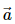makes an acute angle with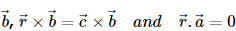, then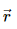is equal to

VITEEE Maths Test - 5 - Question 40

Let a, b and c be distributed non-vegetative numbers. If the vectors aî + aĵ + ck̂, î + k̂ and cî̂ + cĵ̂ + bk̂ lie in a plane, then c is

## VITEEE: Subject Wise and Full Length MOCK Tests

1 videos|3 docs|73 tests
 Use Code STAYHOME200 and get INR 200 additional OFF Use Coupon Code
Information about VITEEE Maths Test - 5 Page
In this test you can find the Exam questions for VITEEE Maths Test - 5 solved & explained in the simplest way possible. Besides giving Questions and answers for VITEEE Maths Test - 5, EduRev gives you an ample number of Online tests for practice

## VITEEE: Subject Wise and Full Length MOCK Tests

1 videos|3 docs|73 tests